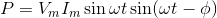# Three Phase Power

It is found that generation of three phase power is more economical than generation of single phase power. In three phase electric power system, the three voltage and current waveform are 120o offset in time in each cycle of power. That means; each voltage waveform has phase difference of 120o to other voltage waveform and each current waveform has phase difference of 120o to other current waveform. Three phase power definition states that in an electrical system, three individual single phase powers are carried out by three separate power circuits. The voltages of these three powers are ideally 120o apart from each other in time-phase. Similarly, the currents of these three powers are also ideally 120o apart from each other. Ideal three phase power system implies balanced system.

A three phase system is said to be unbalanced when either at least one of the three phase voltage is not equal to other or the phase angle between these phases is not exactly equal to 120o.

#### Advantages of Three Phase System

There are many reasons due to which this power is more preferable than single phase power.

1. The single phase power equation isWhich is time dependent function. Whereas three phase power equation isWhich is time independent constant function. Hence the single phase power is pulsating. This generally does not effect the low rating motor but in larger rated motor, it produces excessive vibration. So three phase power is more preferable for high tension power load.
2. The rating of a three phase machine 1.5 times greater than that of same size single phase machine.
3. Single phase induction motor has no starting torque, so we have to provide some auxiliary means of starting, but three phase induction motor is self starting-does not require any auxiliary means.
4. Power factor and efficiency, both are greater in case of three phase system.

## Three Phase Power Equation

For determination, the expression of three phase power equation i.e. for three phase power calculation we have to first consider an ideal situation where the three phase system is balanced. That means voltage and currents in each phase differ from their adjacent phase by 120o as well as the amplitude of each current wave is same and similarly amplitude of each voltage wave is same. Now, the angular difference between voltage and current in each phase of three phase power system is φ.

Then the voltage and current of red phase will berespectively.
The voltage and current of yellow phase will be-respectively.
And the voltage and current of blue phase will be-respectively.
Therefore, the expression instantaneous power in red phase is –Similarly the expression instantaneous power in yellow phase is –Similarly the expression instantaneous power in blue phase is –The total three phase power of the system is summation of the individual power in each phase-The above expression of power shows that the total instantaneous power is constant and equal to three times of the real power per phase. In case of single phase power expression we found that there are both reactive power and active power components, but in case of three phase power expression, the instantaneous power is constant. Actually in three phase system, the reactive power in each individual phase is not zero but sum of them at any instant is zero.Scroll to Top
Scroll to Top